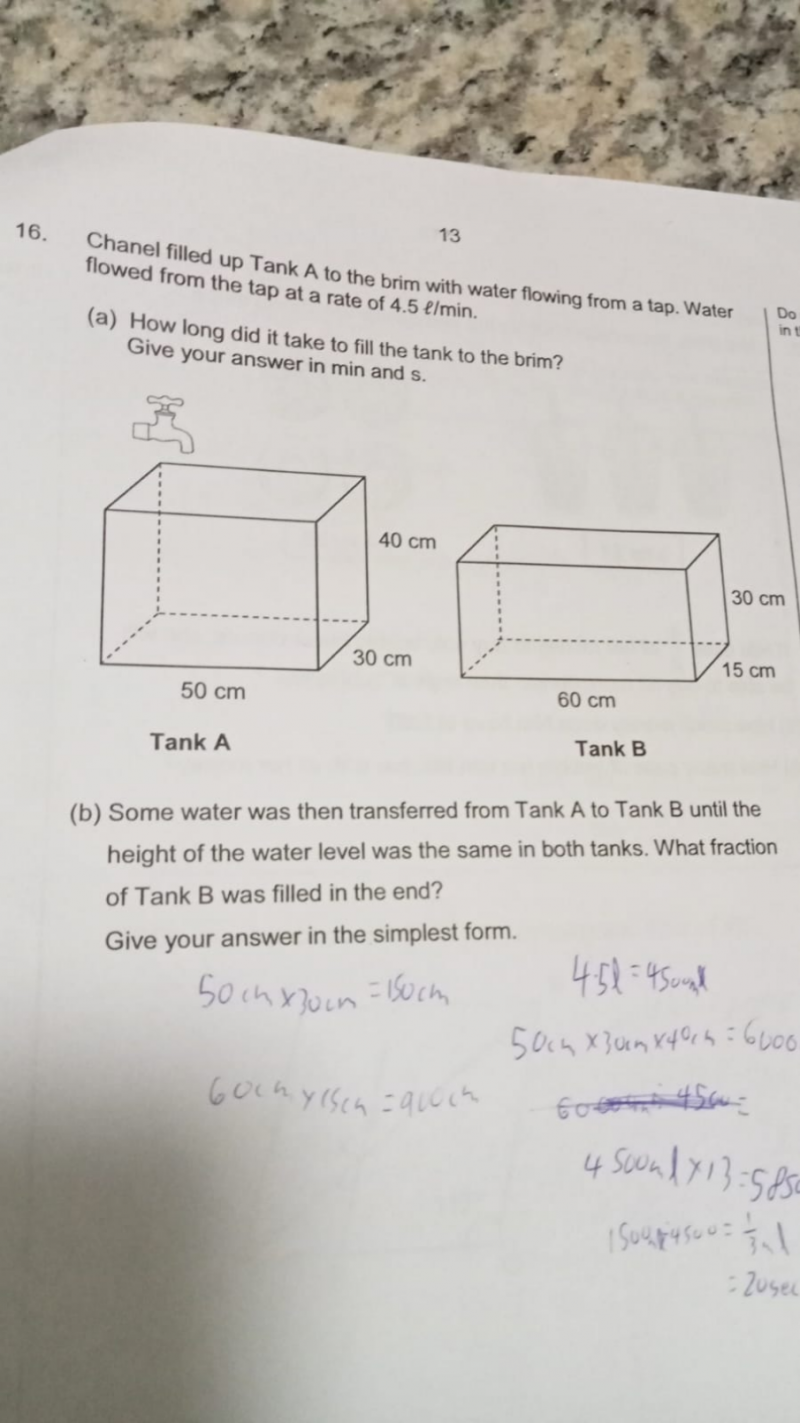# QuestionConfused pls help.

(a) Volume of Tank A = 50x40x30 = 60 000 cm3 = 60 000ml = 60 l

4.5 l —> 1 min

60 l –>  60/4.5 = 13 minutes and 15 seconds

(b) BA of Tank B = 60 x 15 = 900

BA of Tank A = 50 x 30 = 1500

Let h be the height of water of both tanks.

60000 – 1500h = 900h

1500h + 900h = 60000

2400h = 60000

h = 60000/2400 = 25

Fraction of Tank B filled =  25/30 = 5/6

0 Replies 2 Likes ✔Accepted Answer